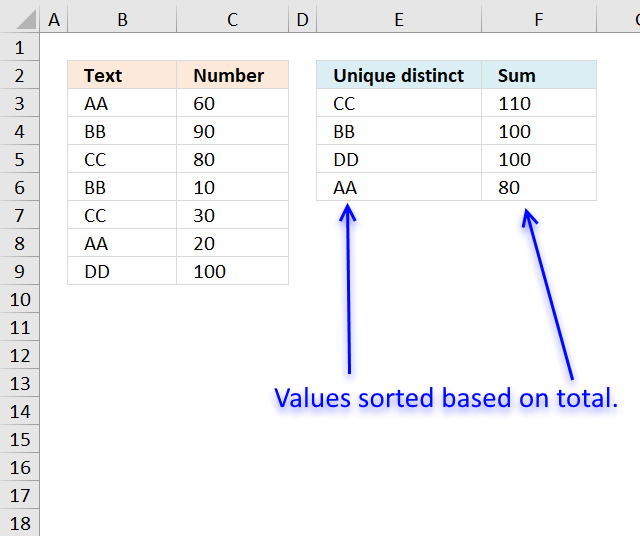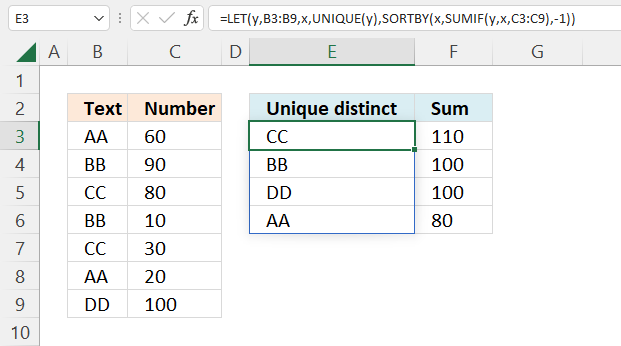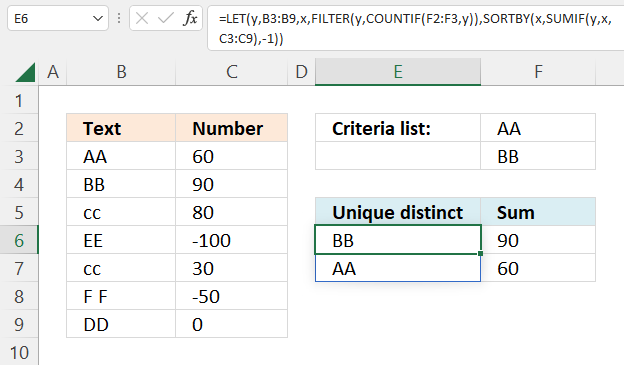Author: Oscar Cronquist Article last updated on October 18, 2022Filtering unique distinct text values and sorting them based on the sum of adjacent values can easily be done by creating a pivot table, and I highly recommend it.

But in this blog post I want to show how to do this using an Excel array formula.

## 1. Extract unique distinct values sorted based on sum of adjacent values - Excel 365This example demonstrates a formula that lists unique distinct values in column B and returns a sorted list based on the totals in column C from large to small.

Value "CC" is displayed in cells C5 and C7, they are 80 and 30 respectively, and the total is 110.

Value "BB" is displayed in cells C4 and C6, they are 90 and 10 respectively, and the total is 100.

Value "DD" is displayed in cell C9, the value is 100, and the total is 100.

Value "AA" is displayed in cells C3 and C7, they are 60 and 20 respectively, and the total is 80.

Excel 365 dynamic array formula:

=LET(y,B3:B9,x,UNIQUE(y),SORTBY(x,SUMIF(y,x,C3:C9),-1))

Formula in cell F3:

=SUMIF(\$B\$3:\$B\$9,E3,\$C\$3:\$C\$9)

### Explaining formula

#### Step 1 - Extract unique distinct values

The UNIQUE function returns a unique or unique distinct list.

Function syntax: UNIQUE(array,[by_col],[exactly_once])

UNIQUE(B3:B9)

becomes

UNIQUE({"AA";"BB";"CC";"BB";"CC";"AA";"DD"})

and returns

{"AA";"BB";"CC";"DD"}.

#### Step 2 - Calculate totals based on the unique list

The SUMIF function sums numerical values based on a condition.

Function syntax: SUMIF(range, criteria, [sum_range])

SUMIF(B3:B9,UNIQUE(B3:B9),C3:C9)

becomes

SUMIF({"AA";"BB";"CC";"BB";"CC";"AA";"DD"}, {"AA";"BB";"CC";"DD"}, {60;90;80;10;30;20;100})

and returns

{80;100;110;100}

#### Step 3 - Sort totals from largest to smallest

The SORTBY function sorts a cell range or array based on values in a corresponding range or array.

Function syntax: SORTBY(array, by_array1, [sort_order1], [by_array2, sort_order2],…)

SORTBY(UNIQUE(B3:B9),SUMIF(B3:B9,UNIQUE(B3:B9),C3:C9),-1)

becomes

SORTBY({"AA";"BB";"CC";"DD"},{60;90;80;10;30;20;100},-1)

and returns

{"CC";"BB";"DD";"AA"}.

#### Step 4 - Shorten the formula

The LET function lets you name intermediate calculation results which can shorten formulas considerably and improve performance.

Function syntax: LET(name1, name_value1, calculation_or_name2, [name_value2, calculation_or_name3...])

SORTBY(UNIQUE(B3:B9),SUMIF(B3:B9,UNIQUE(B3:B9),C3:C9),-1)

y - B3:B9

x - UNIQUE(y)

LET(y,B3:B9,x,UNIQUE(y),SORTBY(x,SUMIF(y,x,C3:C9),-1))

## 2. Extract unique distinct values sorted based on the sum of adjacent values - earlier Excel versionsThis formula is for earlier Excel versions.

Array formula in E2:

=INDEX(\$A\$2:\$A\$8, MATCH(MAX(IF(NOT(COUNTIF(\$D\$1:D1, \$A\$2:\$A\$8)),SUMIF(\$A\$2:\$A\$8, "="&\$A\$2:\$A\$8, \$B\$2:\$B\$8),"")), IF(NOT(COUNTIF(\$D\$1:D1, \$A\$2:\$A\$8)),SUMIF(\$A\$2:\$A\$8, "="&\$A\$2:\$A\$8, \$B\$2:\$B\$8),""), 0))

How to create an array formula

1. Copy above array formula
2. Double press with left mouse button on cell E3
3. Paste array formula
4. Press and hold Ctrl + Shift simultaneouslöy
5. Press Enter

Formula in cell F3:

=SUMIF(\$B\$3:\$B\$9,E3,\$C\$3:\$C\$9)

### Explaining formula in cell E2

#### Step 1 - Count prior values above the current cell

The COUNTIF function counts values based on a condition or criteria, if the number is 0 (zero) then the corresponding value has not yet been displayed.

NOT(COUNTIF(\$D\$1:D1, \$A\$2:\$A\$8))

becomes

NOT(COUNTIF("Unique distinct", {"AA";"BB";"CC";"BB";"CC";"AA";"DD"}))

becomes

NOT({0;0;0;0;0;0;0})

The NOT function returns the boolean opposite to the given argument. The array contains no boolean values, however it does contain their numerical equivavents. TRUE = 1 and FALSE = 0 (zero).

NOT({0;0;0;0;0;0;0})

and returns

{TRUE; TRUE; TRUE; TRUE; TRUE; TRUE; TRUE}.

#### Step 2 - IF TRUE then return the corresponding sum

The IF function returns the total if the boolean value is TRUE. FALSE returns "" (nothing).

IF(NOT(COUNTIF(\$E\$2:E2, \$B\$3:\$B\$9)),SUMIF(\$B\$3:\$B\$9, "="&\$B\$3:\$B\$9, \$C\$3:\$C\$9),"")

becomes

IF({TRUE; TRUE; TRUE; TRUE; TRUE; TRUE; TRUE}, SUMIF(\$B\$3:\$B\$9, "="&\$B\$3:\$B\$9, \$C\$3:\$C\$9),"")

The SUMIF function adds numbers and returns a total based on a condition or criteria.

IF({TRUE; TRUE; TRUE; TRUE; TRUE; TRUE; TRUE}, SUMIF(\$B\$3:\$B\$9, "="&\$B\$3:\$B\$9, \$C\$3:\$C\$9),"")

becomes

IF({TRUE; TRUE; TRUE; TRUE; TRUE; TRUE; TRUE}, {80;100;110;100;110;80;100},"")

and returns

{80;100;110;100;110;80;100}.

#### Step 3 - Get the largest number in array

The MAX function returns the largest number in the array ignoring blanks and text values.

MAX(IF(NOT(COUNTIF(\$E\$2:E2, \$B\$3:\$B\$9)),SUMIF(\$B\$3:\$B\$9, "="&\$B\$3:\$B\$9, \$C\$3:\$C\$9),""))

becomes

MAX({80;100;110;100;110;80;100})

and returns 110.

#### Step 4 - Match number

The MATCH function returns the relative position of a value in a cell range or array.

MATCH(MAX(IF(NOT(COUNTIF(\$E\$2:E2, \$B\$3:\$B\$9)),SUMIF(\$B\$3:\$B\$9, "="&\$B\$3:\$B\$9, \$C\$3:\$C\$9),"")), IF(NOT(COUNTIF(\$E\$2:E2, \$B\$3:\$B\$9)),SUMIF(\$B\$3:\$B\$9, "="&\$B\$3:\$B\$9, \$C\$3:\$C\$9),""), 0)

becomes

MATCH(110, IF(NOT(COUNTIF(\$E\$2:E2, \$B\$3:\$B\$9)),SUMIF(\$B\$3:\$B\$9, "="&\$B\$3:\$B\$9, \$C\$3:\$C\$9),""), 0)

becomes

MATCH(110, {80;100;110;100;110;80;100}, 0)

and returns 3.

#### Step 5 - Get value

The INDEX function returns a value based on row number (and column number if needed)

INDEX(\$A\$2:\$A\$8, MATCH(MAX(IF(NOT(COUNTIF(\$D\$1:D1, \$A\$2:\$A\$8)),SUMIF(\$A\$2:\$A\$8, "="&\$A\$2:\$A\$8, \$B\$2:\$B\$8),"")), IF(NOT(COUNTIF(\$D\$1:D1, \$A\$2:\$A\$8)),SUMIF(\$A\$2:\$A\$8, "="&\$A\$2:\$A\$8, \$B\$2:\$B\$8),""), 0))

becomes

INDEX(\$A\$2:\$A\$8, 3)

and returns "CC" in cell E2.

### Get Excel *.xlsx file

Filter unique distinct list sorted based on sum of adjacent values.xlsx

## 3. Filtering unique distinct text values and sorting them based on the sum of adjacent values and criteria - earlier Excel versionsThis formula is for earlier Excel versions than Excel 365. It extracts values based on the list specified in cells E1 and E2, the formula returns the list sorted based on totals of the adjacent numbers.

Array formula in cell E7:

=INDEX(\$A\$2:\$A\$8, MATCH(MAX(IF(NOT(COUNTIF(\$D\$6:D6, \$A\$2:\$A\$8)+(COUNTIF(\$E\$1:\$E\$2,\$A\$2:\$A\$8)=0)),SUMIF(\$A\$2:\$A\$8, "="&\$A\$2:\$A\$8, \$B\$2:\$B\$8),"")), IF(NOT(COUNTIF(\$D\$6:D6, \$A\$2:\$A\$8)+(COUNTIF(\$E\$1:\$E\$2,\$A\$2:\$A\$8)=0)),SUMIF(\$A\$2:\$A\$8, "="&\$A\$2:\$A\$8, \$B\$2:\$B\$8),""), 0))

Formula in cell E7:

=SUMIF(\$A\$2:\$A\$8,D7,\$B\$2:\$B\$8)

## 4. Filtering unique distinct text values and sorting them based on the sum of adjacent values and criteria - Excel 365This formula is the same as in section 1 with one difference, values must be in the criteria list in order to be displayed.

Excel 365 dynamic array formula in cell E6:

=LET(y,B3:B9,x, UNIQUE(FILTER(y,COUNTIF(F2:F3,y))), SORTBY(x, SUMIF(y,x,C3:C9),-1))

Formula in cell F6:

=SUMIF(\$B\$3:\$B\$9,E6,\$C\$3:\$C\$9)

### Explaining formula

#### Step 1 - Count values based on criteria

The COUNTIF function calculates the number of cells that is equal to a condition.

Function syntax: COUNTIF(range, criteria)

COUNTIF(F2:F3,B3:B9)

becomes

COUNTIF({"AA";"BB"},{"AA";"BB";"cc";"EE";"cc";"F F";"DD"})

and returns

{1; 1; 0; 0; 0; 0; 0}.

#### Step 2 - Filter values based on the count

The FILTER function extracts values/rows based on a condition or criteria.

Function syntax: FILTER(array, include, [if_empty])

FILTER(B3:B9,COUNTIF(F2:F3,B3:B9))

becomes

FILTER({"AA";"BB";"cc";"EE";"cc";"F F";"DD"},{1; 1; 0; 0; 0; 0; 0})

and returns

{"AA";"BB"}.

#### Step 3 - Extract unique distinct values

The UNIQUE function returns a unique or unique distinct list.

Function syntax: UNIQUE(array,[by_col],[exactly_once])

UNIQUE(FILTER(B3:B9,COUNTIF(F2:F3,B3:B9)))

becomes

UNIQUE({"AA";"BB"})

and returns

{"AA";"BB"}.

#### Step 4 - Calculate totals based on the unique list

The SUMIF function sums numerical values based on a condition.

Function syntax: SUMIF(range, criteria, [sum_range])

SUMIF(B3:B9,UNIQUE(FILTER(B3:B9,COUNTIF(F2:F3,B3:B9))),C3:C9)

becomes

SUMIF({"AA";"BB";"cc";"EE";"cc";"F F";"DD"}, {"AA";"BB"}, {60;90;80;-100;30;-50;0})

and returns

{60;90}.

#### Step 5 - Sort totals from largest to smallest

The SORTBY function sorts a cell range or array based on values in a corresponding range or array.

Function syntax: SORTBY(array, by_array1, [sort_order1], [by_array2, sort_order2],…)

SORTBY(UNIQUE(FILTER(B3:B9,COUNTIF(F2:F3,B3:B9))),SUMIF(B3:B9,UNIQUE(FILTER(B3:B9,COUNTIF(F2:F3,B3:B9))),C3:C9),-1)

becomes

SORTBY({"AA";"BB"},{60;90},-1)

and returns

{"BB";"AA"}.

#### Step 6 - Shorten the formula

The LET function lets you name intermediate calculation results which can shorten formulas considerably and improve performance.

Function syntax: LET(name1, name_value1, calculation_or_name2, [name_value2, calculation_or_name3...])

SORTBY(UNIQUE(FILTER(B3:B9,COUNTIF(F2:F3,B3:B9))),SUMIF(B3:B9,UNIQUE(FILTER(B3:B9,COUNTIF(F2:F3,B3:B9))),C3:C9),-1)

y - B3:B9

x - UNIQUE(FILTER(y,COUNTIF(F2:F3,y)))

LET(y,B3:B9,x,UNIQUE(FILTER(y,COUNTIF(F2:F3,y))),SORTBY(x,SUMIF(y,x,C3:C9),-1))